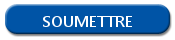# Random samples in R

## Get a random number in R with sample() function

Get a random value in R is very easy, a function is dedicated to this in R. It's the sample() function. At first, you have to specify the possible values. It can be a vector of integer (example: 1:10). If you just specify a vector of possible values, the sample() function return this vector randomly, keeping all the values. For example:

```sample(x = 1:10)
 3 2 8 5 10 9 6 1 7 4```

To select some random values, you have to specify an integer for the size attribute on the sample() function. For example, here is the instruction to get 4 random values of our vector 1:10.

```sample(x = 1:10, size = 4)
 5 9 4 8```

## Get a random textual value in R

As we saw before, the x attribute of the sample() function must be a vector. So, we can use a vector of integers, we can use a vector of character string too. To do that, you must specify a vector of character string and execute the function on this. Here is an example.

```myVector <- c("banana", "apple", "peach", "orange", "strawberry", "apricot")
sample(x = myVector, size = 2)
 "peach" "strawberry"```

## Go further with the sample() function

The sample() function have two more parameters. The replace parameter can be used to find several times the same value, sampling the original vector.

```myVector <- c("banana", "apple", "peach", "orange", "strawberry", "apricot")
sample(x = myVector, size = 3, replace = TRUE)
 " banana " " strawberry " " banana "```

At last, the prob parameter specify a probability for each value of the vector.